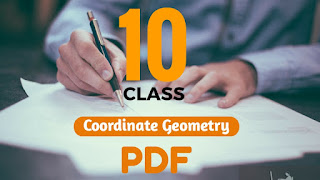# Coordinate Geometry: Class 10 Optional Mathematics SolutionsIn Coordinate Geometry, we learn to locate the position of a certain point with the help of ordered pair of numbered which is also called Coordinates. In this chapter we are going to learn how to find distance between two points, midpoint of two points, slope  and equation of a line.

Coordinate Geometry is also a lengthy chapter of class 10 optional maths. Today I have brought the solution PDF for Coordinate Geometry chapter of class 10 optional mathematics. Due to large number of excercises I have divided the PDF according to exercise number. So in total there are 3 PDF.

Table Of Content :-

You can click on the table of content given above to direct jump on the main exercise PDF if you are facing problem while searching for exercises.

## Chapter - 8  Coordinate Geometry

Before we move to exercises, you need to know some basic formulas which will help you in solving overall problems of Coordinate Geometry.  Please go through the formulas given below:

All Formulas Of  Coordinate Geometry
General Form of a LineAx + By + C = 0
Slope Intercept Form of a Liney = mx + c
Point-Slope Formy − y1= m(x − x1)
The slope of a Line Using Coordinatesm = Δy/Δx = (y− y1)/(x− x1)
The slope of a Line Using General Equationm = −(A/B)
Intercept-Intercept Formx/a + y/b = 1
Distance Formula|P1P2| = √[(x− x1)+ (y− y1)2]
For Parallel Lines,m= m2
For Perpendicular Lines,m1m2 = -1
Midpoint FormulaM (x, y) = [½(x+ x2), ½(y+ y2)]
Angle Formulatan θ = [(m– m2)/ 1 + m1m2]
Area of a Triangle Formula½ |x1(y2−y3)+x2(y3–y1)+x3(y1–y2)|
Distance from a Point to a Lined = [|Ax+ By+ C| / √(A+ B2)]

Now without any delay let's have a look at the solutions of Coordinate geometry chapter.

### Exercise - 8.1 Solutions PDF

NoteScroll the PDF to view all Solution

You are not allowed to post this PDF in any website or social platform without permission.

### Exercise - 8.2 Solutions PDF

NoteScroll the PDF to view all Solution

You are not allowed to post this PDF in any website or social platform without permission.

### Exercise - 8.3 Solutions PDF

NoteScroll the PDF to view all Solution

You are not allowed to post this PDF in any website or social platform without permission.

## Are All the Answers Correct?

Before I answer this question, let me make you clear that I'm neither a teacher nor a topper student. I'm just an average student of my class. So, I can't provide you the guarantee that all the answers are correct. There may be some minor mistakes in answers but the process of solving the problems are correct.

You can recheck the answers before copying, by doing so you can avoid mistakes. If there are mistakes in process of solving the problems then let me know through your comments.

## Is Class 10 Optional Math Guide Helpful For Student?

Of course, Class 10 Optional Mathematics guide is very much helpful for students who wants to score good marks in their examination. Optional mathematics guide contains all the excercise of Optional book with their solutions.

This guide will help you to clear your confusion which you might face while solving optional mathematics questions. You can easily solve the questions by looking the notes given above which are in pdf form.

You have to use this note as a reference for helping you while solving problems. You shouldn't depend fully on this note to complete your excercise, if you do so then it might affect your studies.

## How to secure good marks in Mathematics?

Scoring good marks in optional mathematics is not so easy for an average student.  As I already have mentioned that I'm also an average student as a result I always had a tough time while solving optional mathematics questions. Coordinate Geometry was another confusing chapter for me.

If you want to secure good marks in optional mathematics then you have to work hard. I personally used to go to coaching classes to practise optional mathematics. So I advice you to join coaching classes because it will really help you. You shouldn't only depend on coaching classes, you have to practise personally also.

Disclaimer: This website is made for educational purposes. If you find any content that belongs to you then contact us through the contact form. We will remove that content from our as soon as possible.

Note: If you have any queries or wants to give any suggestion then feel free to comment down

Thanks for your visit. Have a wonderful day 😊
••